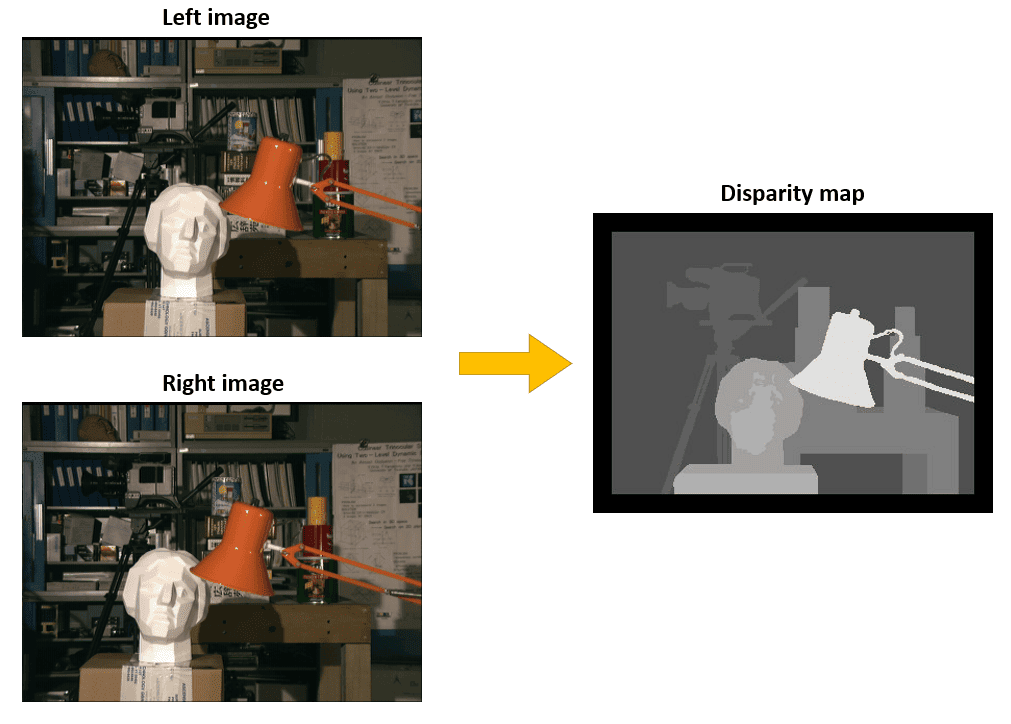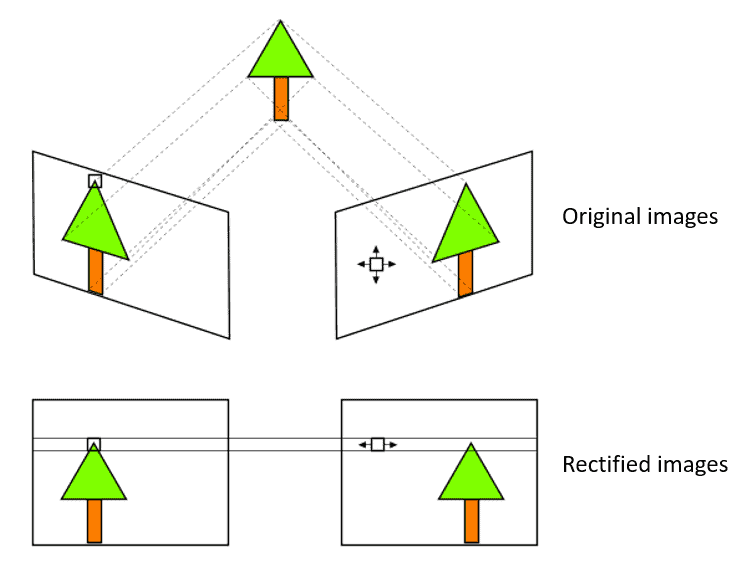## 1. Overview

In this tutorial, we’ll talk about disparity, a fundamental concept in stereo vision. First, we’ll discuss how the disparity map is used in computer vision to reconstruct the 3D structure of a scene from two images. Then we’ll illustrate how to compute the disparity map.

## 2. Stereo Vision

We are all familiar with the perception of depth that our two eyes give us. Computers can emulate this capability with stereo vision, a computer vision technique to estimate the 3D structure of a scene from multiple images. Stereo vision is used in robot navigation applications and in the Advanced Driver Assistance Systems (ADAS).

The basic idea is that two cameras take pictures of the same scene from two different positions, and we aim to estimate the depth for each pixel. This task can be done by finding matching pixels in the two stereo images and knowing the geometric arrangement of the cameras.

## 3. Disparity Map

The disparity is the apparent motion of objects between a pair of stereo images. To experience this, try closing one eye and then rapidly open it while closing the other one. You will note that closer objects will move significantly while objects further away will move very little. This phenomenon is called disparity.

Given a pair of stereo images, to compute the disparity map, we first match every pixel in the left image with its corresponding pixel in the right image. Then we compute the distance for each pair of matching pixels. Finally, the disparity map is obtained by representing such distance values as an intensity image.

Let’s consider, as an example, the following pair of stereo images and the corresponding disparity map.We can see that the objects in the foreground, i.e., the lamp and the statue, appear fairly shifted in the stereo images, and therefore they are marked with brighter pixels in the disparity map. Conversely, the objects in the background have low disparity since their displacement in the stereo images is very small.

As previously observed, the depth is inversely proportional to the disparity. If we know the geometric arrangement of the cameras, then the disparity map can be converted into a depth map using triangulation.

When disparity is near zero, small disparity differences produce large depth differences. However, when disparity is large, small disparity differences do not change the depth significantly. Hence, stereo vision systems have high depth resolution only for objects relatively near the camera.

## 4. Correspondence Problem

To compute the disparity map, we must address the so-called correspondence problem. This task aims at determining the pair of pixels in the stereo images that are projections of the same physical point in space.

An important simplification of the problem can be obtained by the rectification of the stereo images. After this transformation, the corresponding points will lie on the same horizontal line. This reduces the 2D stereo correspondence problem to a 1D problem.

The image rectification process is illustrated in the following figure:A basic approach for finding corresponding pixels is the Block Matching algorithm. It is based on comparing a small window around a point in the first image with multiple small blocks along the same horizontal line in the second image. For each pair of windows, a loss function is computed. The pointin the second image with minimum loss value is the best match for the pointin the first image. Hence, the disparity value at the coordinatewill be.

Two different functions are usually used for finding the matching pixels. The first is the Sum of Absolute Differences (SAD) which computes the sum of elementwise absolute differences of two windowsand, of size, extracted from the two stereo images:The second function is the Sum of Squared Differences (SSD). While the SAD computes a sum of absolute values, the SSD computes the sum of squared differences:In general, SAD is preferable to SSD since it is faster and more robust to noise and outliers.

## 5. Conclusion

In this article, we reviewed the concept of disparity, and we explained its importance in stereo vision. Finally, we illustrated a simple method to compute the disparity map.